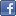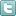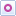EE Times-India > Amplifiers/Converters

Amplifiers/Converters# Rules of thumb: Intrinsic op amp noise

Posted: 26 Oct 2015Print Version

Keywords:operational amplifier  op amp  noise  impedance  resistor

There are many technical documents about noise analysis. Early on in my career I became obsessed with operational amplifier (op amp) noise. Subsequently, I published a series of application notes and a book on the subject. So, why do we need another article? Many engineers don't want to do the full detailed noise analysis and just need some quick rules of thumb to get their designs "in the ball park." For those wanting a quick and simple method to minimise noise, this article is for you.

There are three sources of noise in op amp circuits: op amp voltage noise, op amp current noise, and resistor noise (figure 1). The op amp current noise is translated to noise voltage when it flows through the feedback network and input source impedance.Figure 1: Equivalent noise circuit.

When doing noise calculations, a common approach is to refer all the noise sources to the amplifiers input. You can calculate input-referred noise for feedback resistors by using the equivalent parallel combination of two resistors (Equation 1). You can calculate noise spectral density using Johnson's thermal noise equation (Equation 2 and figure 2 graph), as well as noise from the input resistor (RIN).Figure 2: Noise density vs. resistance.

Combining noise
Using Equation 3, combine noise from the input resistor, RIN, and noise from the equivalent feedback network, REQ. Use this equation whenever you need to combine any random and uncorrelated noise source.Related Articles Editor's Choice
Comment on "Rules of thumb: Intrinsic op amp noi..."

Top Ranked Articles

WebinarsVisit Asia Webinars to learn about the latest in technology and get practical design tips.

Search EE Times India
Services

Go to topConnect on FacebookFollow us on TwitterFollow us on Orkut
﻿

Back to Top